> > Area of a Triangle Using Determinants

# Area of a Triangle Using Determinants

Imagine a triangle with vertices at (x1,y1), (x2,y2), and (x3,y3). If the triangle was a right-angled triangle, it would be pretty easy to compute the area of a triangle by finding one-half the product of the base and the height (area of triangle formula). However, when the triangle is not a right-angled triangle there are multiple different ways to do so. It turns out that the area of triangle formula can also be found using determinants. Let us see in detail how do we go about it.

### Suggested VideosProperties of DeterminantsApplications of DeterminantsMinors and Cofactors_H## Area of Triangle Formula Using Determinants

In earlier classes, we have studied that the area of a triangle whose vertices are  (x1, y1), (x2, y2) and (x3, y3), is given by the expression $$\frac{1}{2} [x1(y2–y3) + x2 (y3–y1) + x3 (y1–y2)]$$. Now this expression can be written in the form of a determinant as### Points to be Noted

• Since the area is a positive quantity, we always take the absolute value of the determinant in (1).
• If the area is given, uses both positive and negative values of the determinant for calculation.
• The area of the triangle formed by three collinear points is zero.

Example: Find the area of the triangle whose vertices are (3, 8), (– 4, 2) and (5, 1).
Solution: The area of the triangle is given by= $$\frac{1}{2} [ 3(2 –1) – 8(– 4 – 5) + 1(– 4 –10)]$$
= $$\frac{1}{2} ( 3 + 72 – 14 ) = 61/2$$## Derivation of the Area of Triangle Formula• We know that the Area of Rectangle can be written as follows• Area of Triangle A: Technically, each of those distances should be the absolute value of the difference. But the problem is much easier to work without the absolute values.• Area of Triangle B: Realize, however, as if the points don’t lie in the same positions (point 2 is both the rightmost and the uppermost), that the area found using these formulas will be negative.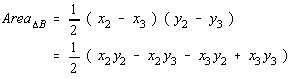• Area of Triangle C: For that reason, caution should be exerted to always make the final answer non-negative. The area of a triangle, after all, can’t be negative.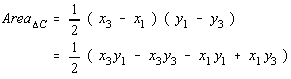### Let’s add the areas of the three outside triangles together.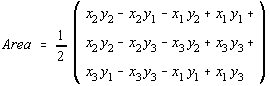Simplifying further,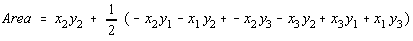Now, to subtract the areas of the three triangles from the area of the rectangle.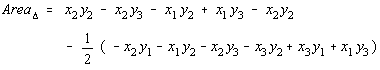Simplifying further,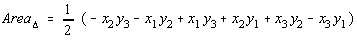Let’s regroup those terms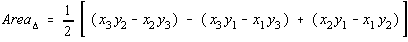………….. (1)

Now, consider the determinant formed by placing the x-coordinates in the first column, the y-coordinates in the second column, and the constant 1 in the last column.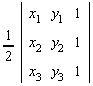Let’s evaluate the determinant by expanding along the 3rd column.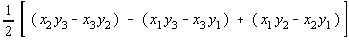…………… (2)

On comparing both (1) and (2) we notice that the area of the triangle differs only in sign. The reason for this is because of the order the points were chosen in. If the points were chosen to be points 1, 2, and 3 in a different order, then the determinant would change only in sign.

Signup and access 1000+ hours of video lectures of Class 12 for free.

## Solved Examples on Area of Triangle Formula

Question 1: If the lines p1x + q1y = 1, p2x + q2y = 1 and p3x + q3y = 1 be concurrent, then the points (p1,q1), (p2,q2) and (p3,q3) ,

1. form scalene triangle
2. form equilateral triangle
3. are collinear
4. form a right-angled triangle

Answer : p1q11, p2q21 p3q31. Given lines are concurrent $$\begin{vmatrix} p_{1} & q_{1} & 1\\ p_{2} & q_{2} & 1 \\ p_{3} & q_{3} & 1\end{vmatrix} = 0$$

The left-hand side of the above equation is also equal to twice the area of a triangle with coordinates (p1,q1)(p2,q2)(p3,q3). From the area of triangle formula, and since the area is equal to zero, (p1,q1)(p2,q2)(p3,q3are collinear.

Question 2: Explain the formula of finding the area of triangle?

Answer: In order to find the area of a triangle, one must multiply the base by the height. Afterward, one must divide it by 2. The division by 2 comes is because one can divide a parallelogram into 2 triangles.

Question 3: How can one find the area of a right triangle?

Answer: In order to find the area of any right triangle, multiplication of the lengths of the two sides must take place. These two sides are perpendicular to each other. Afterward, one must take half of that.

Question 4: Define the area of a triangle?

Answer: One can define the area of a triangle as the total space enclosed by a given triangle.

Question 5: How can one calculate the area of a scalene triangle?

Answer: A scalene triangle is one which all three sides of different lengths. The area of a scalene triangle that has a base b and height h is given by 1/2 bh. If one knows the lengths of all three sides, one can find area by making use of the Heron’s Formula without the need to find the height.

Share with friends

## Customize your course in 30 seconds

##### Which class are you in?
5th
6th
7th
8th
9th
10th
11th
12th
Get ready for all-new Live Classes!
Now learn Live with India's best teachers. Join courses with the best schedule and enjoy fun and interactive classes.Ashhar Firdausi
IIT Roorkee
BiologyDr. Nazma Shaik
VTU
ChemistryGaurav Tiwari
APJAKTU
Physics
Get Started

## Browse

##### Determinants1 Followers

Most reacted comment
1 Comment authorsRecent comment authors
Subscribe
Notify ofGuest
jannat

Is determinant available just for square matrix?Guest
Stephen

Yes, it is only defined for square matrices.Guest
J. A. Zahálka

It actually is. It is equal to zero for all non-square matrices.

## Question Mark?

Have a doubt at 3 am? Our experts are available 24x7. Connect with a tutor instantly and get your concepts cleared in less than 3 steps.

No thanks.